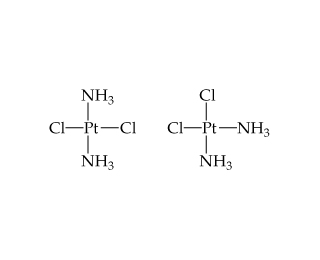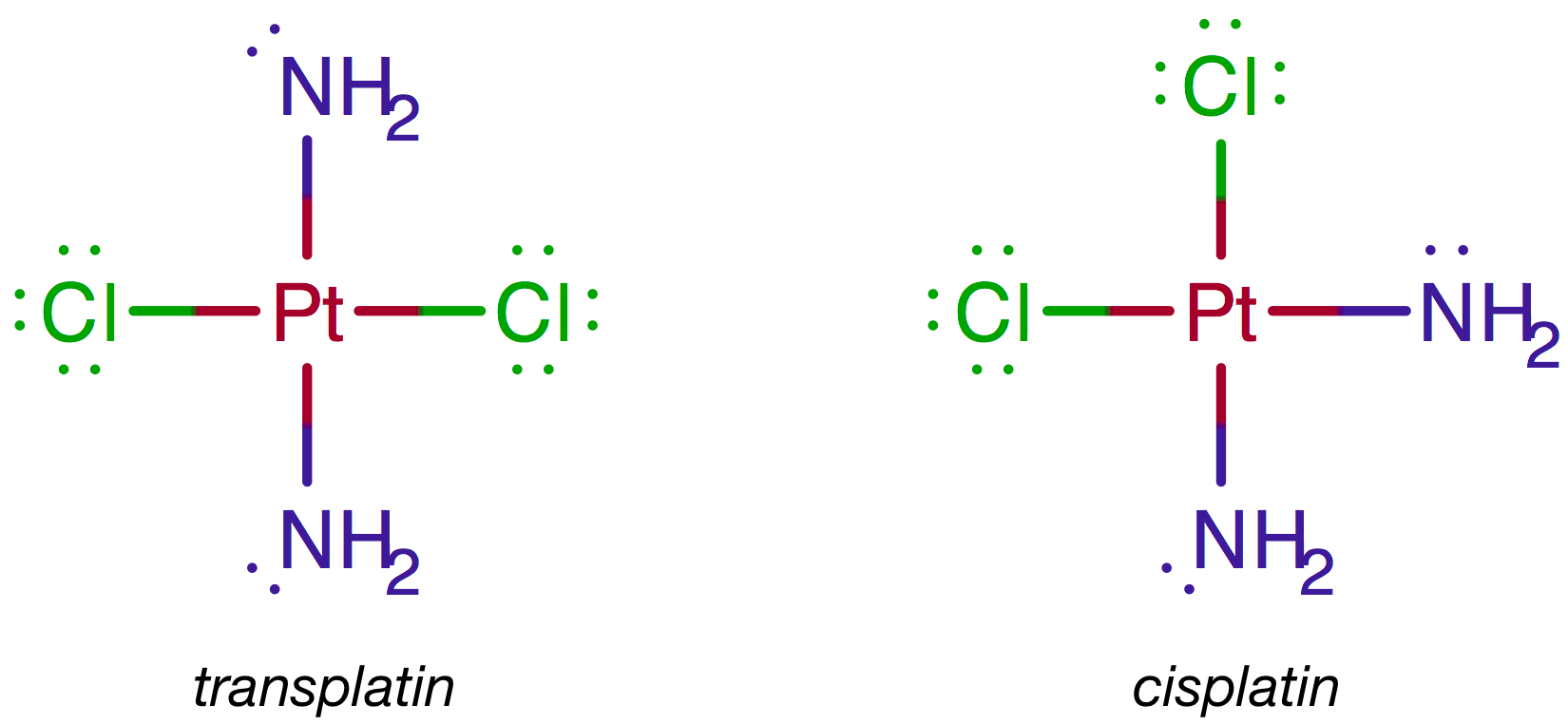Chemistry Practice Problems Dipole Moment Practice Problems Solution: There are two compounds of the formula Pt(NH3 )2 C...

# Solution: There are two compounds of the formula Pt(NH3 )2 Cl2 as shown below. The compound on the right is called cisplatin, and the compound on the left is called transplatin.Which compound has a nonzero dipole moment?

###### Problem

There are two compounds of the formula Pt(NH3 )2 Cl2 as shown below. The compound on the right is called cisplatin, and the compound on the left is called transplatin.Which compound has a nonzero dipole moment?

###### Solution

There are two possible Lewis structures for Pt(NH3)2Cl2:Platinum (EN = 2.28) is less electronegative than nitrogen (EN = 3.04) and chlorine (EN = 3.16) so the dipole moments point away from Pt.View Complete Written Solution

Dipole Moment

Dipole Moment

#### Q. Which of the following incorrectly shows the bond polarity? Show the correct bond polarity for those that are incorrect.a. 8+H—F8-  b. 8+Cl—I8- c. 8+S...

Solved • Mon Nov 26 2018 16:33:45 GMT-0500 (EST)

Dipole Moment

#### Q. Does the molecule BF2Cl have a dipole moment?

Solved • Mon Nov 12 2018 15:50:15 GMT-0500 (EST)

Dipole Moment

#### Q. Ammonia reacts with boron trifluoride to form a stable compound.The B-N bond is obviously more polar than the C-C bond. Draw the charge distribution y...

Solved • Mon Nov 12 2018 15:50:14 GMT-0500 (EST)

Dipole Moment

#### Q. Calculate the dipole moment for HF (bond length 0.917 Å), assuming that the bond is completely ionic.

Solved • Mon Nov 12 2018 15:49:59 GMT-0500 (EST)